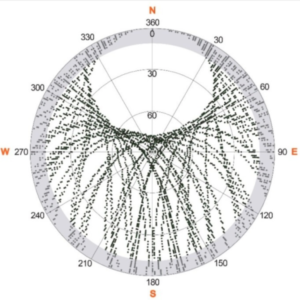Skip to content

What can I say, GPS people like to known where the satellites are with respect to their receivers. This is typically called a “sky plot” or azimuth-elevation angle plot. The means you have to know what I mean by these two quantities. Azimuth is just the “map-view” angle with respect to north, i.e. north is 0, east is 90, south is 180 …  Elevation angle is the angle with the respect to the local horizon. Here is a nice one from Koch et al.Matlab code for sky plot.

The azimuth angles are shown around the largest circle at 30 degree increments. You can also see concentric circles, and those designate the elevation angles (0,30,60).  90 degrees (i.e. right above the GPS antenna) is in the center. What you can see immediately is at this site (in Switzerland), there is a “hole” to the North. There is a similar hole to the south in the  southern hemisphere. Note also the gray zone. This is the region where elevation angles are less than 10 degrees, and these data are often discarded (or not even collected) by geodesists. But these data are great for reflections!

I almost never use sky plots in GPS reflections because I want to get a feel for the spatial footprint of the reflection.  Later we will talk about Fresnel zones, but for now, I will just show you what a reflection point looks like if I assume that an antenna is 1.8 meters above the reflecting zone.